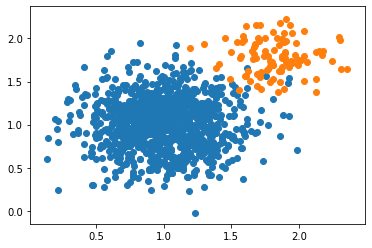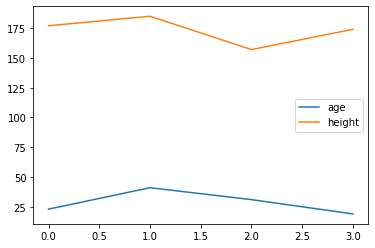# Practical Lab: Python Primer¶

In this notebook, we give a brief overview of Python and Pandas, looking at the kind of tooling that you will need for the Cyber Security Analytics module. Most of the details here should already be familiar to you - hence why the detail is limited in part - so you should be able to read through and understand the operations being performed in this notebook. If there are aspects that look unfamiliar, play around with the notebook, change the code and see what happens - notebooks are a fantastic resource for modifying and trying out techniques.

In :
# First let's import some key data attributes

import numpy as np
import pandas as pd
import matplotlib.pyplot as plt
import seaborn as sns


To begin - let's show a very quick example of generating some dataset and plotting this. Do not worry if you do not understand the commands being used - you will do soon. For now, this is just to show how quickly we can generate this using Python.

In :
a = np.random.normal(1.0, 0.3, [1000,1000])
b = np.random.normal(1.8, 0.2, [100,100])

In :
plt.scatter(a, a)
plt.scatter(b, b)

Out:
<matplotlib.collections.PathCollection at 0x7fa094a77a10># Basic Operations in Python¶

In :
number = 1
text = 'hello_everyone'
l = [1,2,3,4,5]
d = {'name':'bob', 'value':100}


Basic variables in Python include numerical values (integers, floats, doubles, etc.) and string values (text). Basic data structures include lists and dictionaries.

• Lists are essentially like arrays. We can create a dynamic group of (mixed) variables. We can append and remove from this list, and we can access elements from the list.
• Dictionaries are like objects. We can create name-value pairs to reference attributes that make up an object (e.g., properties of a car).
• We can create lists of dictionaries, and we can have lists within dictionaries. We can also have a list of lists (nested lists), and we can have dictionaries as values in a dictionary.

## Let's walk through some Python examples¶

First let's look at some basics of Python and get use to manipulating data using the built in variable types.

In :
# First some simple variables

an_integer = 12
a_floating_point_number = 18.4732

In :
# We can do some simple maths on these variables and see the output

an_integer + a_floating_point_number

Out:
30.4732
In :
# We can also write functions to do simple maths

def multiply(number1, number2):
return number1 * number2

multiply(an_integer, a_floating_point_number)

Out:
221.67839999999998
In :
# We can also create text variables just as easily

a_string = 'Hello there!'
print (a_string)

Hello there!

In :
# We can do some simple manipulation of text

my_name = 'Phil'
message = a_string + ' My name is ' + my_name
print (message)

# Including spliting sentences to corrupt a message
imposter_name = 'Dave'
s_m = message.split(" ")
new_message = ' '.join(s_m[:-1]) + ' ' + imposter_name
print (new_message)

Hello there! My name is Phil
Hello there! My name is Dave

In :
# We also have lists of data that can sort variables
fruits = ['apple','banana','orange','lemon']
print (fruits)
# We can access sets of variables using indexes
print (fruits[0:2])
print (fruits[:-1])
# We can append items to the list, and remove items from the list
fruits.append('mango')
print (fruits)
fruits.remove('banana')
print (fruits)

['apple', 'banana', 'orange', 'lemon']
['apple', 'banana']
['apple', 'banana', 'orange']
['apple', 'banana', 'orange', 'lemon', 'mango']
['apple', 'orange', 'lemon', 'mango']

In :
# We can also create dictionary objects

person = {}
person['name'] = 'bob'
person['age'] = 23
person['height'] = 185
person['email'] = 'bob@bobmail.com'
print (person)

{'name': 'bob', 'age': 23, 'height': 185, 'email': 'bob@bobmail.com'}

In :
# Like earlier, we could use a function to create 'person' objects
people = []

def create_person(name, age, height, email):
global people
new_person = {'name':name,
'age':age,
'height':height,
'email':email}
people.append(new_person)

create_person('bob', 23, 177, 'bob@bobmail.com')
create_person('john', 41, 185, 'john@johnmail.com')
create_person('sophie', 31, 157, 'sophie@sophiemail.com')
create_person('wendy', 19, 174, 'wendy@wendymail.com')

# Here we store our person objects in our people list
# to make a group of 'persons' - a.k.a. people!
print (people)

[{'name': 'bob', 'age': 23, 'height': 177, 'email': 'bob@bobmail.com'}, {'name': 'john', 'age': 41, 'height': 185, 'email': 'john@johnmail.com'}, {'name': 'sophie', 'age': 31, 'height': 157, 'email': 'sophie@sophiemail.com'}, {'name': 'wendy', 'age': 19, 'height': 174, 'email': 'wendy@wendymail.com'}]


## Introducing data science libraries¶

We have covered a lot very quickly here. You've now already used the main built in variables of Python, that allow you to store numerical and text data, and the data structures such as lists (which are essentially arrays), and dictionaries (which are essentially objects). Let's now explore this deeper by introducing some of the data science libraries.

In :
# We can import libraries using the following
import numpy as np
import pandas as pd
import matplotlib.pyplot as plt

In :
# Our people dictionary is difficult for us to read clearly
# Pandas DataFrames help manipulate tabular data like this very easily

data = pd.DataFrame(people)
data

Out:
name age height email
0 bob 23 177 bob@bobmail.com
1 john 41 185 john@johnmail.com
2 sophie 31 157 sophie@sophiemail.com
3 wendy 19 174 wendy@wendymail.com
In :
# We can access individual columns of the data now
data['age']

Out:
0    23
1    41
2    31
3    19
Name: age, dtype: int64
In :
# Who is the tallest of the users? Let's find out
data[data['height'] == np.max(data['height'])]

Out:
name age height email
1 john 41 185 john@johnmail.com
In :
# Who is the shortest of the users? Let's find out
data[data['height'] == np.min(data['height'])]

Out:
name age height email
2 sophie 31 157 sophie@sophiemail.com
In :
# What if we want to plot this data quickly?
data.plot()
plt.show()# What next?¶

Spend some time researching into Pandas, Matplotlib, and Numpy - these are core to manipulating numerical and tabular data, and then being able to visualize the results. There are many great examples online of getting started with these libraries. E.g., (Free e-book 'A Whirlwind Tour of Python': http://www.oreilly.com/programming/free/a-whirlwind-tour-of-python.csp)

In [ ]: